2015-01-19 21:18:57 Bright_Geek 阅读数 1029
• ###### 卷积网络中特征的N种使用方式

卷积神经网络视频教程，该课程以卷积神经网络处理图像为例，探讨了卷积得到的特征图（feature maps）的特性和运用，展示了特征在数据重建、图像生成、对抗样本、相似图查找等等领域的广泛运用，并辅以代码示例，帮助学员更好地理解深度学习和传统机器学习，乃至传统计算机视觉的关系。

1631 人正在学习 去看看 CSDN讲师

OpenCV卷积函数：

void cvFilter2D( const CvArr*  src,

CvArr*             dst,

const CvMat* kernel,

CvPoint           anchor=cvPoint(-1,-1)

);

#include <cv.h>
#include <highgui.h>
using namespace std;

int main()
{
IplImage* src=cvCreateImage(cvSize(700,400),src1->depth,src1->nChannels);
cvResize(src1,src);
IplImage* dst=cvCloneImage(src);

/*Kernal的两种建立方式*/
//1.cvmSet不支持分数！！！
CvMat *k1;
k1=cvCreateMat(3,3,CV_32FC1);

/*cvmSet(k1,0,0,1/16);
cvmSet(k1,0,1,2/16);
cvmSet(k1,0,2,1/16);
cvmSet(k1,1,0,2/16);
cvmSet(k1,1,1,4/16);
cvmSet(k1,1,2,2/16);
cvmSet(k1,2,0,1/16);
cvmSet(k1,2,1,2/16);
cvmSet(k1,2,2,1/16);*/

cvmSet(k1,0,0,0.0625);
cvmSet(k1,0,1,0.125);
cvmSet(k1,0,2,0.0625);
cvmSet(k1,1,0,0.125);
cvmSet(k1,1,1,0.25);
cvmSet(k1,1,2,0.125);
cvmSet(k1,2,0,0.0625);
cvmSet(k1,2,1,0.125);
cvmSet(k1,2,2,0.0625);

//这种方法很方便！！
/*float k1={1,2,1,
2,4,2,
1,2,1};
for(int i=0;i<9;i++)
{
k1[i]=(float)k1[i]/16;
}

CvMat k2=cvMat(3,3,CV_32F,&k1);*/

cvFilter2D(src,dst,k1);//卷积操作

cvNamedWindow("src");
cvNamedWindow("dst");
cvShowImage("src",src);
cvShowImage("dst",dst);
cvWaitKey(0);
cvReleaseImage(&src);
cvReleaseImage(&dst);
cvDestroyAllWindows();

return 0;
}2018-11-14 16:07:10 u012005313 阅读数 789
• ###### 卷积网络中特征的N种使用方式

卷积神经网络视频教程，该课程以卷积神经网络处理图像为例，探讨了卷积得到的特征图（feature maps）的特性和运用，展示了特征在数据重建、图像生成、对抗样本、相似图查找等等领域的广泛运用，并辅以代码示例，帮助学员更好地理解深度学习和传统机器学习，乃至传统计算机视觉的关系。

1631 人正在学习 去看看 CSDN讲师

# 图像卷积概念

Convolution

Kernel (image processing)

# 图像卷积数学公式

$h[x,y]=f[x,y]\ast g[x,y]=\sum_{n_{1}=-\infty}^{\infty}\sum_{n_{2}=-\infty}^{\infty}f(n_1, n_2)\cdot g(x-n_{1},y-n_{2})$

## 图形化表示

$y(t)=(f\ast g)(t)=\sum_{\tau =-\infty}^{\infty}f(\tau )g(t-\tau )d\tau$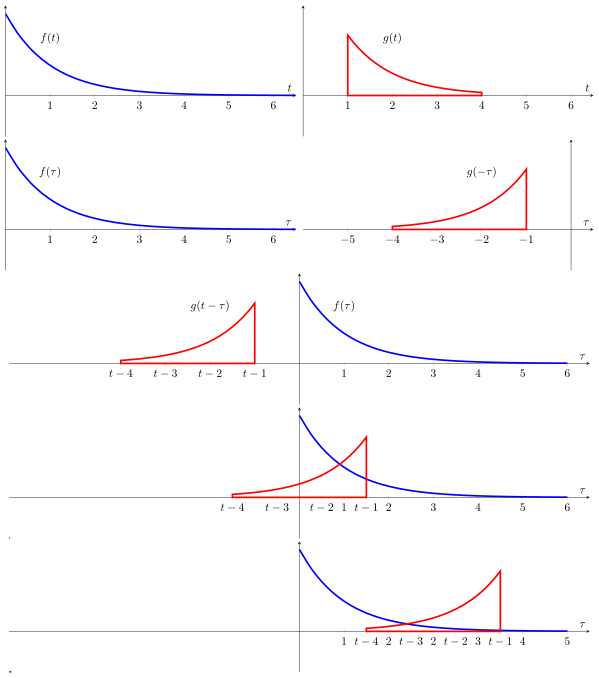• 首先将函数$g(\tau)$反射为$g(-\tau)$，相当于$g(\tau)$沿$y$轴翻转
• 再对函数$g(-\tau)$添加一个时间偏移量$t$，它允许函数$g(t-\tau)$沿着$\tau$轴移动
• 变量$t$每增加1，表示函数$g(t-\tau)$向左移动一步
• 计算t遍历$-\infty$$\infty$的过程中，函数$f(\tau)$$g(t-\tau)$的重叠面积

### 举例

$h(0)=\cdot =1\cdot 1=1$
$h(1)=[1,2]\cdot [3,1]=1\cdot 3+2\cdot 1=3+2=5$
$h(2)=[1,2,3]\cdot [2,3,1]=1\cdot 2 +2\cdot 3+3\cdot 1=2+6+3=11$
$h(3)=[2,3,4]\cdot [2,3,1]=2\cdot 2+3\cdot 3+4\cdot 1=4+9+4=17$
$h(4)=[3,4]\cdot [2,3]=3\cdot 2+4\cdot 3=6+12=18$
$h(5)=\cdot =4\cdot 2=8$
$h(x)=[1, 5, 11, 17, 18, 8]$

• flip the mask (horizontally and vertically) only once(水平和垂直翻转掩模一次)
• slide the mask onto the image(在图像上滑动掩模)
• multiply the corresponding elements and then add them(将相应的元素相乘，然后求和)
• repeat this procedure until all values of the image has been calculated(重复这一过程，直到所有图像值均已被计算)

## 卷积核为啥要翻转?

LTILSI中，信号在时间和空间中移动不改变其特性，不断有信号随时间移动和系统产生响应，某一时刻的输出（即卷积输出）不仅包括当前信号的响应，还有之前信号的残留，所以是累加的，转换卷积核是为了计算这一过程。

### 如何翻转？

$\begin{bmatrix} 1& 2& 3\\ 4& 5& 6\\ 7& 8& 9 \end{bmatrix}\frac{180^{^{\circ}}}{\Rightarrow} \begin{bmatrix} 9& 8& 7\\ 6& 5& 4\\ 3& 3& 1 \end{bmatrix}$

$\begin{bmatrix} 1& 2& 3\\ 4& 5& 6\\ 7& 8& 9 \end{bmatrix}\Rightarrow \begin{bmatrix} 3& 2& 1\\ 6& 5& 4\\ 9& 8& 7 \end{bmatrix}\Rightarrow \begin{bmatrix} 9& 8& 7\\ 6& 5& 4\\ 3& 2& 1 \end{bmatrix}$

## 边界填充方式

1. 扩展（extend）：扩展最近邻的像素点，即扩展图像边界
2. 环绕（wrap）：假设图像是首尾相接的，即使用图像相反方向的像素值
3. 镜像（mirror）：超出边界多少个像素，就向图像内部读取对应位置的像素
4. 固定值：超出图像边界的像素取固定值，默认为0

## 卷积操作和傅里叶变换

• 在空间域执行卷积操作，相当于在频率域执行滤波操作(乘法操作)
• 在频率域执行卷积操作，相当于在空间域执行滤波操作(乘法操作)

$f\ast g=DFT(f)\cdot DFT(g)$
$DFT(f)\ast DFT(g)=f\cdot g$

# 图像卷积算法实现

Signal processing (scipy.signal) Convolution

Multi-dimensional image processing (scipy.ndimage)

Python有多个包（Numpy/Scipy/OpenCV）提供了卷积操作

## Numpy

numpy.convolve

Numpy包提供了方法convolve，用于计算一维线性卷积

• full：默认方式，计算所有重叠的面积
• same：返回和最大数组一样长度的数组。从卷积核锚点位置开始计算
• valid：仅计算完全重叠的面积

import numpy as np

a = np.array([1, 2, 3, 4])
v = np.array([1, 3, 2])
full = np.convolve(a, v)
same = np.convolve(a, v, 'same')
valid = np.convolve(a, v, 'valid')

[ 1  5 11 17 18  8]
[ 5 11 17 18]
[11 17]


## Scipy.signal

scipy.signal包提供了好几种方法来计算卷积：

convolve(in1, in2[, mode, method])
correlate(in1, in2[, mode, method])
fftconvolve(in1, in2[, mode])
convolve2d(in1, in2[, mode, boundary, fillvalue])
correlate2d(in1, in2[, mode, boundary, …])
sepfir2d(input, hrow, hcol)
choose_conv_method(in1, in2[, mode, measure])


### signal.convolve2d()

scipy.signal.convolve2d

convolve2d(in1, in2, mode='full', boundary='fill', fillvalue=0)


in1in2的维数大小相同

• full：输出全离散线性卷积（默认）
• valid：输出不依赖于零填充的卷积结果
• same：输出大小和in1一样，卷积核锚点对齐图像处理的结果

3种填充模式：

1. fill：默认方式，使用fillvalue的值进行填充
2. wrap：环形填充
3. symm：镜像模式

### signal.convolve()

convolve(in1, in2, mode='full', method='auto')


convolve用于计算N维数组的卷积，除了可以设定模式外，还可以选择计算方法：

1. direct：直接按照卷积的定义进行加权求和计算
2. fft：使用快速傅里叶变换（fft）进行卷积操作
3. auto：默认方式，估计计算时间，选择直接计算还是快速傅里叶方法

## Signal.ndimage

signal.ndiamge包提供了多个卷积函数：

convolve(input, weights[, output, mode, …])
convolve1d(input, weights[, axis, output, …])
correlate(input, weights[, output, mode, …])
correlate1d(input, weights[, axis, output, …])


## OpenCV

filter2D()

BorderTypes

Depth combinations

OpenCV提供了一个2维滤波器filter2D

def filter2D(src, ddepth, kernel, dst=None, anchor=None, delta=None, borderType=None)

src：输入图像
dst：相同大小和通道的结果图像
ddepth：结果图像深度，看Depth combinations
kernel：卷积核，一个单通道浮点矩阵
anchor：卷积核锚点位置，默认值（-1,-1），表示在核中心
delta：额外值
borderType：边界填充像素方式，参考BorderTypes


import cv2 as cv
import numpy as np
import matplotlib.pyplot as plt

def realize_sobel():
ddepth = cv.CV_16S

kernel = np.array([[-1, 0, 1], [-2, 0, 2], [-1, 0, 1]], dtype=np.float)
res_x = cv.filter2D(gray, ddepth, kernel)
abs_res_x = cv.convertScaleAbs(res_x)

sobel_x = cv.Sobel(gray, ddepth, 1, 0, ksize=3)
abs_sobel_x = cv.convertScaleAbs(sobel_x)

plt.figure(figsize=(10, 5))  # 设置窗口大小
plt.suptitle('sobel')  # 图片名称
plt.subplot(1, 2, 1)
plt.title('filter2D_x')
plt.imshow(abs_res_x, cmap='gray'), plt.axis('off')
plt.subplot(1, 2, 2)
plt.title('sobel_x')
plt.imshow(abs_sobel_x, cmap='gray'), plt.axis('off')
plt.savefig('./sobel_x.png')  # 保存图像
plt.show()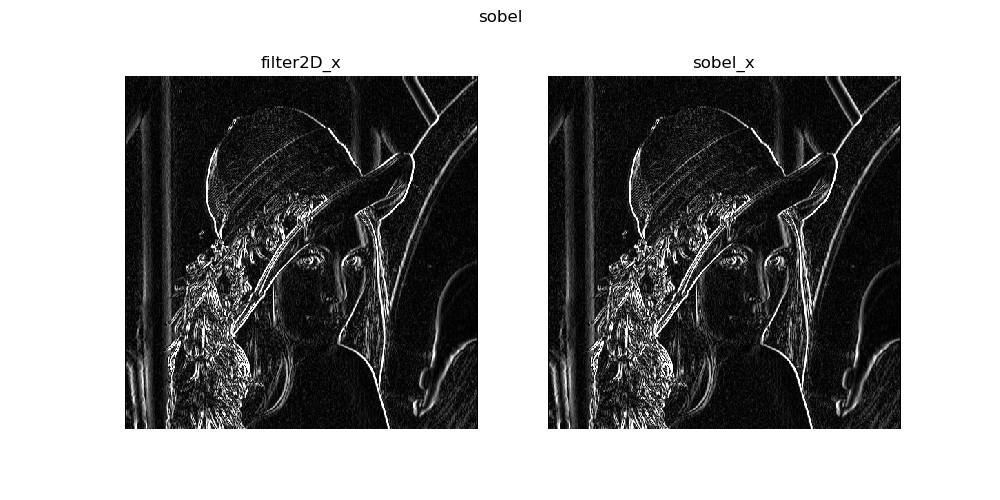2018-06-27 16:30:41 heiheiya 阅读数 409
• ###### 卷积网络中特征的N种使用方式

卷积神经网络视频教程，该课程以卷积神经网络处理图像为例，探讨了卷积得到的特征图（feature maps）的特性和运用，展示了特征在数据重建、图像生成、对抗样本、相似图查找等等领域的广泛运用，并辅以代码示例，帮助学员更好地理解深度学习和传统机器学习，乃至传统计算机视觉的关系。

1631 人正在学习 去看看 CSDN讲师

# 定义

卷积是图像处理中很常见的操作，它通过计算原图像和一个卷积核的加权和来对图像进行滤波。根据卷积核的不同，可以完成对图像的模糊、锐化、降噪、边缘检测等其他功能。

离散二维卷积公式可以表示为，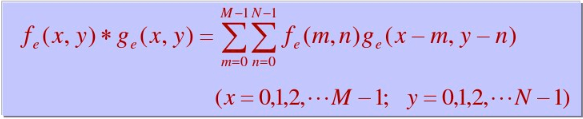上述公式中，f即表示原图像，g表示卷积核。卷积核的中心总是对准当前计算的像素点。

不同的卷积核可以完成不同的图像处理效果。

# 一、 平滑

图像平滑可以衰减图像中的高频分量。最常见的平滑是领域均值滤波，它的卷积核的每一个值都等于1，比如一个3x3的卷积核是这样的，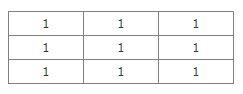最终该点像素值等于该领域内像素的均值。

// maximum size supported by this shaderconst
int MaxKernelSize = 25;
// array of offsets for accessing the base imageuniform
vec2 Offset[MaxKernelSize];
// size of kernel (width * height) for this executionuniform
int KernelSize;
// final scaling valueuniform
vec4 ScaleFactor; // image to be convolveduniform
sampler2D BaseImage;
void main(){
int i;
vec4 sum = vec4(0.0);
for (i = 0; i < KernelSize; i++)
sum += texture2D(BaseImage,gl_TexCoord.st + Offset[i]);
gl_FragColor = sum * ScaleFactor;}

图像平滑通常被用来做图像降噪，这对稠密的或者纯色的区域表现很好，但是它有一个副作用就是会滤掉高频分量，也就是会影响到图像边缘信息。如果对越接近中心的值给予越来的权重，就能在降噪的同时保持好边缘信息，比如高斯滤波器。但是随着卷积核的增大，对纹理的读取次数以核的维度的平方增加，核越大，计算效率越低。但是对于像高斯这样的滤波器，一个widthxheight大小的卷积核，可以分成水平widthx1和垂直1xheight两个一维的卷积。虽然这样每个像素会增加一次写操作，但是纹理的读取却从widthxheight减少到width+height次。

# 二、 边缘检测

使用拉普拉斯卷积核可以实现边缘检测，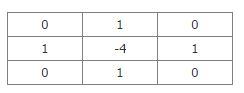最终效果如下所示。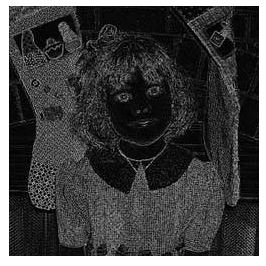# 三、 锐化

完成锐化一个简单常用的方法是把边缘检测的结果与原图像相加。为控制锐化的程度，需要给边缘检测的图像乘以一个权重。锐化时使用的是负的拉普拉斯卷积核。

// maximum size supported by this shaderconst
int MaxKernelSize = 25;
// array of offsets for accessing the base imageuniform
vec2 Offset[MaxKernelSize];
// size of kernel (width * height) for this executionuniform
int KernelSize;
// value for each location in the convolution kerneluniform
vec4 KernelValue[MaxKernelSize];
// scaling factor for edge imageuniform
vec4 ScaleFactor;
// image to be convolveduniform
sampler2D BaseImage;
void main(){
int i;
vec4 sum = vec4(0.0);
for (i = 0; i < KernelSize; i++)    {
vec4 tmp = texture2D(BaseImage,
gl_TexCoord.st + Offset[i]);
sum += tmp * KernelValue[i];   }
vec4 baseColor = texture2D(BaseImage,vec2(gl_TexCoord));
gl_FragColor = ScaleFactor * sum + baseColor;}


最后出来的效果如下图。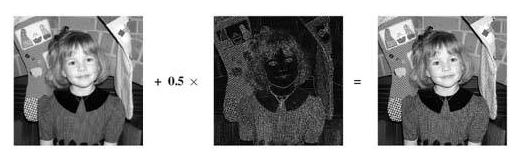2019-08-02 13:55:46 Unicornshawn 阅读数 310
• ###### 卷积网络中特征的N种使用方式

卷积神经网络视频教程，该课程以卷积神经网络处理图像为例，探讨了卷积得到的特征图（feature maps）的特性和运用，展示了特征在数据重建、图像生成、对抗样本、相似图查找等等领域的广泛运用，并辅以代码示例，帮助学员更好地理解深度学习和传统机器学习，乃至传统计算机视觉的关系。

1631 人正在学习 去看看 CSDN讲师

### 有趣的图像处理

在这里我们将会简单了解到什么是卷积操作，并使用卷积对图像进行处理。

## 1.预备知识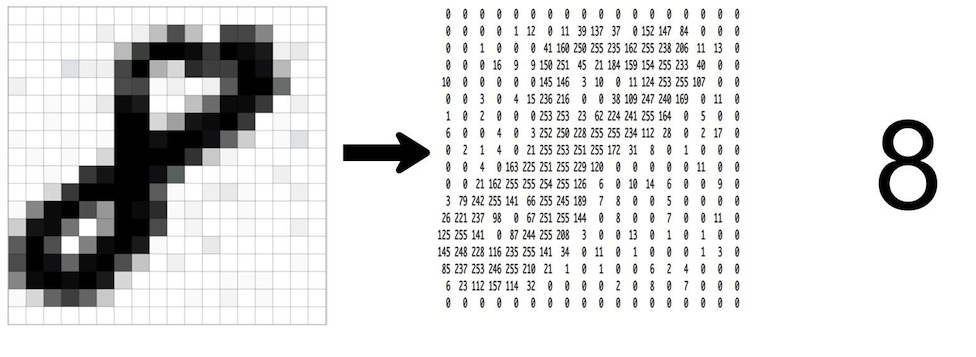我们需要知道的是，在计算机中，图像是由很多个像素点组合而成，我们对图像的卷积处理其实就是对这些像素点进行一定的运算处理。知道这一点我们就可以开始以下的学习了。

## 2.卷积操作

在图像处理中，卷积操作指的就是使用一个卷积核(kernel)对一张图像中的每个像素进行一系列操作。卷积核通常是一个四方形网格结构(例如2x2、3x3的方形区域)，该区域内每个方格都有一个权重值。当对图像中的某个像素进行卷积时，我们会把卷积核的中心放置于该像素上，翻转核之后再依次计算核中每个元素和其覆盖的图像像素值的乘积并求和，得到的结果就是该位置的新像素值。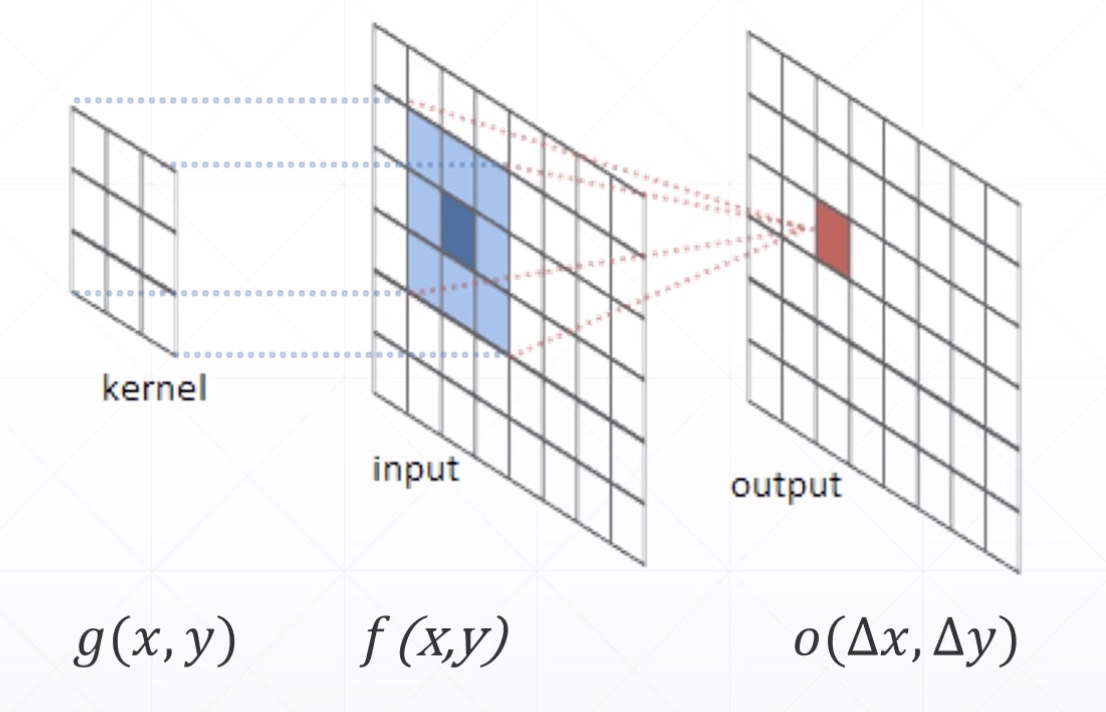动态演示如下：动态演示中我们会发现6 * 6的图经过卷积处理后我们得到了4 * 4的图，这是因为我们在处理图像边界的时候有两种选择。其一，我们可以把最外围的像素“丢弃”掉，这是最直接的；其二，我们在最外围再添加一圈0像素，这样我们就可以同样使用卷积得到边界像素。

## 3.卷积的应用

在了解了卷积操作后，我们来实际地使用卷积处理原图，得到有趣的图片吧！

（1）锐化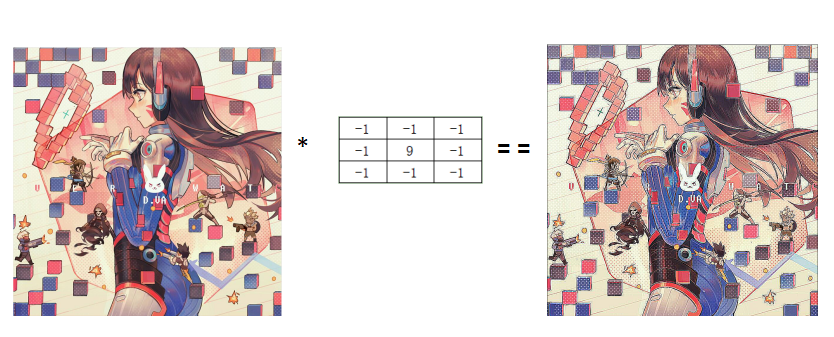（2）浮雕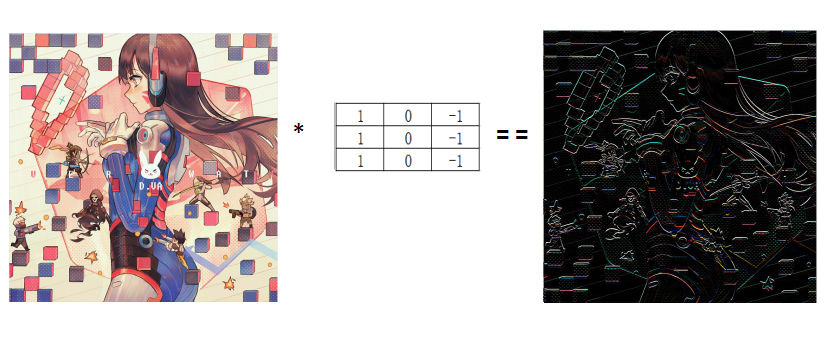（3）强调边缘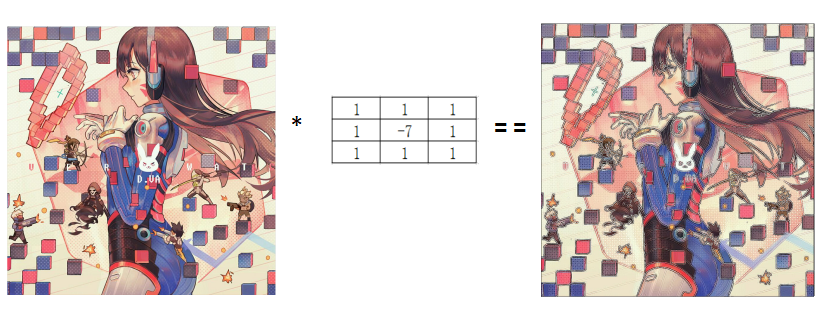代码如下：
（注：以下代码还实现了图像的灰白化、黑白化、镜像等，有兴趣可以参考了解一下）

package imagePro;

import java.awt.Graphics;

import javax.swing.JFrame;

public class Pro {
Graphics g;//画布
public void showUI(){
//窗口基本属性
JFrame jf=new JFrame();
jf.setSize(1100, 1100);
jf.setDefaultCloseOperation(jf.DISPOSE_ON_CLOSE);
jf.setLocationRelativeTo(null);

//监听器对象
ImgListener lis=new ImgListener();

//菜单栏

//菜单选项

//子选项

//添加监听器

//菜单添加选项

//添加菜单
//设置菜单栏

jf.setVisible(true);//窗体可见
g=jf.getGraphics();//获取窗体的画布
lis.setGraphics(g);
}
public static void main(String[] args){
Pro pro=new Pro();
pro.showUI();
}
}


监听器类

package imagePro;

import java.awt.Color;
import java.awt.Graphics;
import java.awt.event.ActionEvent;
import java.awt.event.ActionListener;
import java.awt.image.BufferedImage;
import java.io.File;
import java.io.IOException;

import javax.imageio.ImageIO;

public class ImgListener implements ActionListener{

BufferedImage image;//图片
BufferedImage img;//临时图片
BufferedImage img_1;//图片黑白化
Graphics temp_g;//临时画布

Graphics g;
public void actionPerformed(ActionEvent e) {
String str=e.getActionCommand();
System.out.println(str);
if("加载图片".equals(str)){
}
if("绘制图片".equals(str)){
draw();
}
if("灰度化".equals(str)){
huidu();
}
if("黑白化".equals(str)){
heibai();
}
if("镜像".equals(str)){
mirror();
}
if("高斯模糊".equals(str)){
Guess();
}
if("浮雕".equals(str)){
fudiao();
}
if("锐化".equals(str)){
ruihua();
}
if("强调边缘".equals(str)){
edge();
}

}
try {
} catch (IOException e) {
e.printStackTrace();
}
}
public void draw(){
g.drawImage(image, 100, 100, null);
}
public void huidu(){
img=new BufferedImage(image.getWidth(), image.getHeight(), BufferedImage.TYPE_INT_RGB);
temp_g=img.getGraphics();
//分别获取R/G/B值
for(int i=0;i<image.getWidth();i++){
for(int j=0;j<image.getHeight();j++){
int RGB=image.getRGB(i, j);
int R=(RGB>>16)&0xff;
int G=(RGB>>8)&0xff;
int B=RGB&0xff;
//绘制该点
int gray=(int)(0.3*R+0.59*G+0.11*B);
temp_g.setColor(new Color(gray,gray,gray));
temp_g.drawLine(i, j, i, j);
}
}
g.drawImage(img, 100, 100, null);
}
public void heibai(){
img=new BufferedImage(image.getWidth(),image.getHeight(),BufferedImage.TYPE_INT_RGB);
temp_g=img.getGraphics();
//分别获取R/G/B值
for(int i=0;i<image.getWidth();i++){
for(int j=0;j<image.getHeight();j++){
int RGB=image.getRGB(i, j);
int R=(RGB>>16)&0xff;
int G=(RGB>>8)&0xff;
int B=RGB&0xff;
//绘制该点
int ave=(R+G+B)/3;
if(ave<78){
temp_g.setColor(Color.BLACK);
}else
temp_g.setColor(Color.WHITE);

temp_g.drawLine(i, j, i, j);
}
}
img_1=img;
g.drawImage(img, 100, 100, null);
}
public void mirror(){
img=new BufferedImage(image.getWidth(),image.getHeight(),BufferedImage.TYPE_INT_RGB);
temp_g=img.getGraphics();

//分别获取R/G/B值
for(int i=0;i<image.getWidth();i++){
for(int j=0;j<image.getHeight();j++){
int RGB=image.getRGB(i, j);
int R=(RGB>>16)&0xff;
int G=(RGB>>8)&0xff;
int B=RGB&0xff;
//绘制该点
temp_g.setColor(new Color(R,G,B));
temp_g.drawLine(image.getWidth()-i-1, j, image.getWidth()-i-1, j);
}
}
g.drawImage(img, 100, 100, null);
}
public void Guess(){
double value[]={0.075,0.124,0.075,0.124,0.204,0.124,0.075,0.124,0.075};
drawPic(img, value);
}
private void fudiao() {
double value[]={1,0,-1,1,0,-1,1,0,-1};
drawPic(img, value);
}
private void edge() {
double value[]={1,1,1,1,-7,1,1,1,1};
drawPic(img, value);
}
private void ruihua() {
double value[]={-1,-1,-1,-1,9,-1,-1,-1,-1};
drawPic(img, value);
}
public void drawPic(BufferedImage img,double[] value){
img=new BufferedImage(image.getWidth(),image.getHeight(),BufferedImage.TYPE_INT_RGB);
temp_g=img.getGraphics();
int pix[]=new int;
for(int i=1;i<img.getWidth()-1;i++){
for(int j=1;j<img.getHeight()-1;j++){
temp_g.setColor(changePix(pix, value));//设置该点的颜色
temp_g.drawLine(i, j, i, j);
}
}
g.drawImage(img, 100, 100, null);
}
public void readPix(BufferedImage img,int x,int y,int[]pix){
int start=0;
for(int i=x-1;i<x+2;i++){
for(int j=y-1;j<y+2;j++){
pix[start++]=img.getRGB(i, j);//3*3的数组转化为1*9的数组
}
}
}
public Color changePix(int[] pix,double[] value){
double R=0;
double G=0;
double B=0;
//分别获取R、G、B的值
for(int i=0;i<pix.length;i++){
R+=((pix[i]>>16)&0xff)*value[i];
G+=((pix[i]>>8)&0xff)*value[i];
B+=(pix[i]&0xff)*value[i];
}
if(R>255)R=255;
if(R<0)R=0;
if(G>255)G=255;
if(G<0)G=0;
if(B>255)B=255;
if(B<0)B=0;
return new Color((int)R,(int)G,(int)B);
}
public void setGraphics(Graphics g){
this.g=g;
}
}


参考：（图像处理基本知识）https://zhuanlan.zhihu.com/p/55013828

卷积操作 图像处理 相关内容

2017-09-29 23:25:39 qq_35144795 阅读数 4168
• ###### 卷积网络中特征的N种使用方式

卷积神经网络视频教程，该课程以卷积神经网络处理图像为例，探讨了卷积得到的特征图（feature maps）的特性和运用，展示了特征在数据重建、图像生成、对抗样本、相似图查找等等领域的广泛运用，并辅以代码示例，帮助学员更好地理解深度学习和传统机器学习，乃至传统计算机视觉的关系。

1631 人正在学习 去看看 CSDN讲师

# 一、 什么是卷积？

在图像处理中，卷积操作指的是使用一个卷积核对图像中的每个像素进行一系列操作。
卷积核（算子）是用来做图像处理时的矩阵,图像处理时也称为掩膜，是与原图像做运算的参数。卷积核通常是一个四方形的网格结构（例如3*3的矩阵或像素区域），该区域上每个方格都有一个权重值。
使用卷积进行计算时，需要将卷积核的中心放置在要计算的像素上，一次计算核中每个元素和其覆盖的图像像素值的乘积并求和，得到的结构就是该位置的新像素值。
以下两个算子中演示了具体的卷积计算过程。

# 二、 相关算子

定义：，其中ｈ称为相关核(Kernel).

步骤：

• 1）滑动核，使其中心位于输入图像g的（i，j）像素上
• 2）利用上式求和，得到输出图像的（i，j）像素值
• 3）充分上面操纵，直到求出输出图像的所有像素值

【例】
原始像素矩阵为：

17234101124561218171319258142021215162239

卷积模板h为：

834159672

计算输出图像的（2，4）元素=1*8+8*1+15*6+7*3+14*5+16*7+13*4+20*9+22*2=585
如图所示：# 三、 卷积算子

定义：步骤：
- 1）将核围绕中心旋转180度
- 2）滑动核，使其中心位于输入图像g的（i，j）像素上
- 3）利用上式求和，得到输出图像的（i，j）像素值
- 4）充分上面操纵，直到求出输出图像的所有像素值
例：计算输出图像的（2，4）元素=1*2+8*9+15*4+7*7+14*5+16*3+13*6+20*1+22*8=575
如图所示：# 四、 边缘效应

当对图像边缘的进行滤波时，核的一部分会位于图像边缘外面。常用的策略包括：
- 1）使用常数填充：imfilter默认用0填充，这会造成处理后的图像边缘是黑色的。
- 2）复制边缘像素：I3 = imfilter(I,h,’replicate’);# 五、 常用的卷积核及其用途

• 1）低通滤波器（常用于计算模糊后的效果）
1/91/91/91/91/91/91/91/91/9

1/101/101/101/102/101/101/101/101/10

1/162/161/162/164/162/161/162/161/16
• 2）高斯滤波器（常用于计算高斯模糊后的效果）
高斯模糊的卷积核也是一个正方形的滤波核，其中每个元素通过以下公式计算得出：
G(x,y)=12πσ2ex2+y22σ2
该公式中σ是标准方差（一般取值为1），x和y分别对应了当前位置到卷积核中心的整数距离。通过这个公式，就可以计算出高斯核中每个位置对应的值。为了保证滤波后的图像不会变暗，需要对高斯核中的权重进行归一化。

• 3）边缘检测（常用于计算图像边缘或者说梯度值）

101040101

# 六、 一个例子——使用卷积实现模糊效果

我们将对下面这张图进行模糊处理：[numthreads(32,32,1)]
void Dim_Main (uint3 id : SV_DispatchThreadID)
{

float sumR = 0;
float sumG = 0;
float sumB = 0;
float sumA = 0;
for (int i = -1; i <= 1; i++)
{
for (int j = -1; j <= 1; j++)
{
sumR += texBuffer[(id.x+i)*texWidth+(id.y+j)].r * convolutionTempBuffer[(i+1)*3+(j+1)];
sumG += texBuffer[(id.x+i)*texWidth+(id.y+j)].g * convolutionTempBuffer[(i+1)*3+(j+1)];
sumB += texBuffer[(id.x+i)*texWidth+(id.y+j)].b * convolutionTempBuffer[(i+1)*3+(j+1)];
sumA += texBuffer[(id.x+i)*texWidth+(id.y+j)].a * convolutionTempBuffer[(i+1)*3+(j+1)];
}
}

texBuffer[id.x*texWidth+id.y].r = sumR;
texBuffer[id.x*texWidth+id.y].g = sumG;
texBuffer[id.x*texWidth+id.y].b = sumB;
texBuffer[id.x*texWidth+id.y].a = sumA;

Result[id.xy] = float4(sumR, sumG, sumB, sumA);
}1234567891011121314151617181920212223242526

效果如图所示：图中可以明显的看到左右两边有明显的黑色线条，原图中是没有这样的黑色的，产生这种效果的原因是本文中之前提到过的边缘效应。下面我将修改一部分代码去除边缘效应带来的影响，这里使用的是相邻像素的值方法。
代码如下：

[numthreads(32,32,1)]
void Dim_Main (uint3 id : SV_DispatchThreadID)
{

float sumR = 0;
float sumG = 0;
float sumB = 0;
float sumA = 0;
for (int i = -1; i <= 1; i++)
{
for (int j = -1; j <= 1; j++)
{
if((id.x+i)*texWidth+(id.y+j)>texWidth*texWidth-1)
{
sumR += texBuffer[(id.x+i)*texWidth+(id.y+j)-texWidth].r * convolutionTempBuffer[(i+1)*3+(j+1)];
sumG += texBuffer[(id.x+i)*texWidth+(id.y+j)-texWidth].g * convolutionTempBuffer[(i+1)*3+(j+1)];
sumB += texBuffer[(id.x+i)*texWidth+(id.y+j)-texWidth].b * convolutionTempBuffer[(i+1)*3+(j+1)];
sumA += texBuffer[(id.x+i)*texWidth+(id.y+j)-texWidth].a * convolutionTempBuffer[(i+1)*3+(j+1)];
}

sumR += texBuffer[(id.x+i)*texWidth+(id.y+j)].r * convolutionTempBuffer[(i+1)*3+(j+1)];
sumG += texBuffer[(id.x+i)*texWidth+(id.y+j)].g * convolutionTempBuffer[(i+1)*3+(j+1)];
sumB += texBuffer[(id.x+i)*texWidth+(id.y+j)].b * convolutionTempBuffer[(i+1)*3+(j+1)];
sumA += texBuffer[(id.x+i)*texWidth+(id.y+j)].a * convolutionTempBuffer[(i+1)*3+(j+1)];
}
}

texBuffer[id.x*texWidth+id.y].r = sumR;
texBuffer[id.x*texWidth+id.y].g = sumG;
texBuffer[id.x*texWidth+id.y].b = sumB;
texBuffer[id.x*texWidth+id.y].a = sumA;

Result[id.xy] = float4(sumR, sumG, sumB, sumA);
}12345678910111213141516171819202122232425262728293031323334

效果如图所示：可以看到，图中左边的黑色线条已经被滤除，右边也可以采用类似的方法来剔除。实际使用中，也可以根据情况使用纯色来做剔除，这样可以节省部分效率，如下图中我使用的是纯白色来剔除边缘效应。卷积操作 图像处理 相关内容

阅读数 130

博文 来自： qq_33668060

阅读数 4203

博文 来自： computerme

阅读数 23290

博文 来自： xueyedie1234

阅读数 14382

博文 来自： HXG2006

阅读数 433

博文 来自： qq_35789421
没有更多推荐了，返回首页